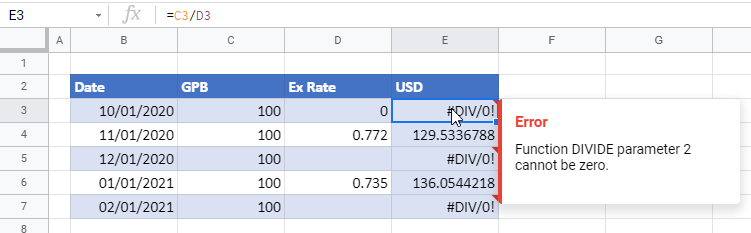# How to Divide Cells / Columns – Excel & Google Sheets

This tutorial will demonstrate how to divide cells and columns in Excel and Google Sheets.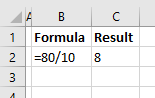## The Divide Symbol

The divide symbol in Excel is the forward slash on the keyboard (/). This is the same as using the division sign (÷) in mathematics. When you divide two numbers in Excel, start with an equal sign (=), which will create a formula. Then type in the first number (the number you want to divide), followed by the forward slash, and then the number you wish to divide by.

``=80/10``

The result of this formula would then be 8.

## Divide With a Cell Reference and a Constant

For greater flexibility in your formula, use the reference of a cell as the number to be divided and a constant as the number to divide by.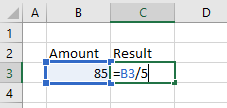To divide B3 by 5, type the formula:

``=B3/5``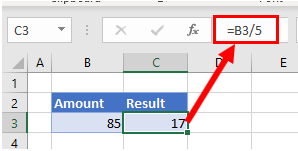Then copy this formula down the column to the rows below. The constant number (5) will remain the same but the cell address for each row will change according to the row you are in due to Relative Cell referencing.

## Divide a Column With Cell References

You can also use a cell reference for the number to divide by.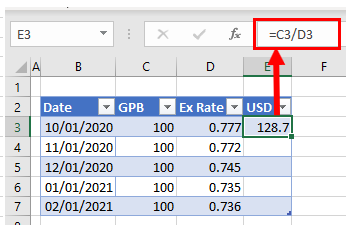To divide cell C3 by cell D3, type the formula:

``=C3/D3``

Since the formula uses cell addresses, you can then copy this formula down to the rest of the rows in the table.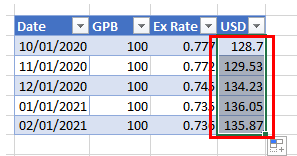As the formula uses Relative Cell addresses, the formula will change according to the row it is copied down to.

Note: that you can also divide numbers in Excel by using the QUOTIENT Function.

## Divide a Column With Paste Special

You can divide a column of numbers by a divisor, and return the result as a number within the same cell.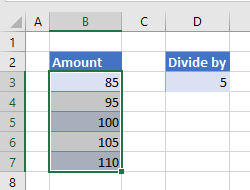1. Select the divisor (in this case, 5) and in the Ribbon, go to Home > Copy, or press CTRL + C.
1. Highlight the cells to be divided (in this case B3:B7).
1. In the Ribbon, go to Home > Paste > Paste Special.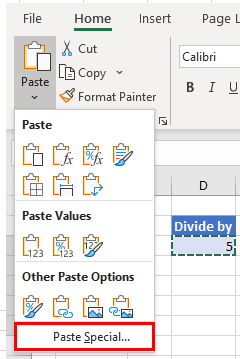1. In the Paste Special dialog box, select Divide and then click OK.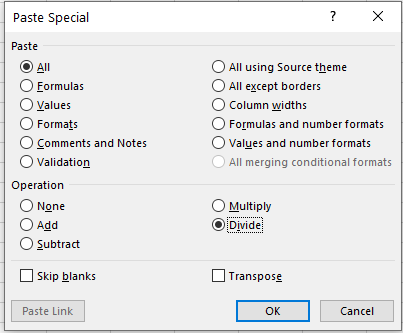The values in the highlighted cells will be divided by the divisor that was copied and the result will be returned in the same location as the highlighted cells. In other words, you will overwrite your original values with the new divided values; cell references are not used in this this method of calculation.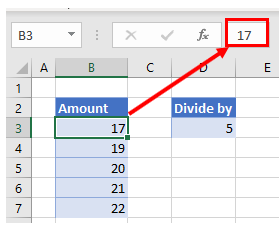## The #DIV/0! Error

If you try and divide a number by zero, you will get an error as there is no answer when a number is divided by zero.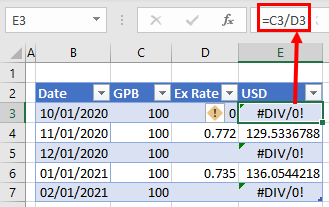You will also get an error if you try and divide a number by a blank cell.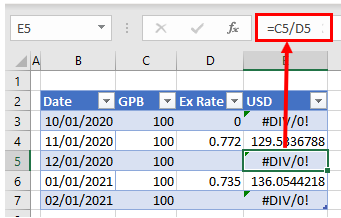To prevent this from happening, you can use the IFERROR Function in your formula.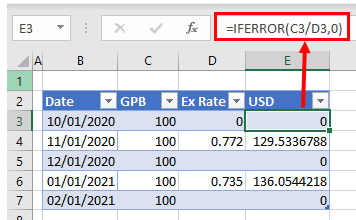Using the methods above, you can also add, subtract, or multiply cells and columns in Excel.

### Easy Excel Automation